# Introduction to pyshtools¶

pyshtools is a Python package that provides functions for working with spherical harmonics. These routines can be used to perform spherical harmonic transforms and reconstructions, rotations of data expressed in spherical harmonics, and multitaper spectral analyses on the sphere. The base functions are fast routines written in Fortran 95 from the SHTOOLS package, and pyshtools provides easy access to these routines by use of Python-wrapper functions and a compact class interface.

To get started, import the standard matplotlib library for graphics, numpy for mathematical extensions to Python, and pyshtools:

In :
from __future__ import print_function # only necessary if using Python 2.x
%matplotlib inline

import matplotlib.pyplot as plt
import numpy as np
import pyshtools


The pyshtools package contains several classes, including SHCoeffs, SHGrid, and SHWindow, a subpackage shtools that contains the Python-wrapped Fortran functions, and a series of subpackages that contain both native python routines and refernces to routines in shtools (constant, legendre, expand, shio, spectralanalysis, rotate, gravmag, and util). The classes SHCoeffs, SHGrid, and SHWindow bundle the vast majority of pyshtools functionality, and in this notebook we will demonstrate how to use the classes related to grids and coefficients.

pyshtools can also define matplotlib configuration variables for creating publication quality graphics. These can be set using the function figstyle and by specifing the relative width of the image with respect to a journal page. If necessary, one can also specify optional parameters for the resolution of the screen, the maximum width of the image, and the default image aspect ratio.

In :
pyshtools.utils.figstyle(rel_width=0.75)
%config InlineBackend.figure_format = 'retina'  # if you are not using a retina display, comment this line!


## Grid and Coefficient classes¶

Let's start by first creating a power spectrum (i.e., the total power per degree) that follows a power law with exponent -2, up to and including degree 100. To avoid a division by zero, we will set the degree 0 term to zero:

In :
degrees = np.arange(101, dtype=float)
degrees = np.inf
power = degrees**(-2)


Next, we will create a random realization of spherical harmonic coefficients whose expected power is given by the spectrum we just created:

In :
clm = pyshtools.SHCoeffs.from_random(power, seed=12345)


This creates a new class instance of SHCoeffs that contains several attributes and methods. For reproducibility, the optional parameter seed specifies the seed of the numpy random number generator. By default, pyshtools assumes that the coefficients are real and that they are normalized using the '4pi' convention exlcuding the Condon-Shortley phase factor. This is the standard normalization in Geodesy and many fields of geophysics and spectral analysis. Other normalizations can be specified explicitly by specifying the optional parameter normalization, which can be '4pi', 'ortho', 'schmidt', or 'unnorm'. The Condon-Shortley phase can be included by setting the optional parameter csphase to -1, and if you wanted complex coefficients, you could set kind='complex'.

from_random() is just one way to create a set of spherical harmonic coefficients. The other constructor methods are from_file() to read the coefficients from an shtools or npy formatted file, from_zeros() if you just want all the coefficients to be set to zero, and from_array() if you already have a numpy array of the coefficients.

Next, let's calculate the power spectrum and plot it. pyshtools provides a built in plotting function to do this, and as we will see below, the power spectrum can also be returned as a numpy array using the spectrum() method.

In :
fig, ax = clm.plot_spectrum(show=False)    # show=False is used to avoid a warning when plotting in inline mode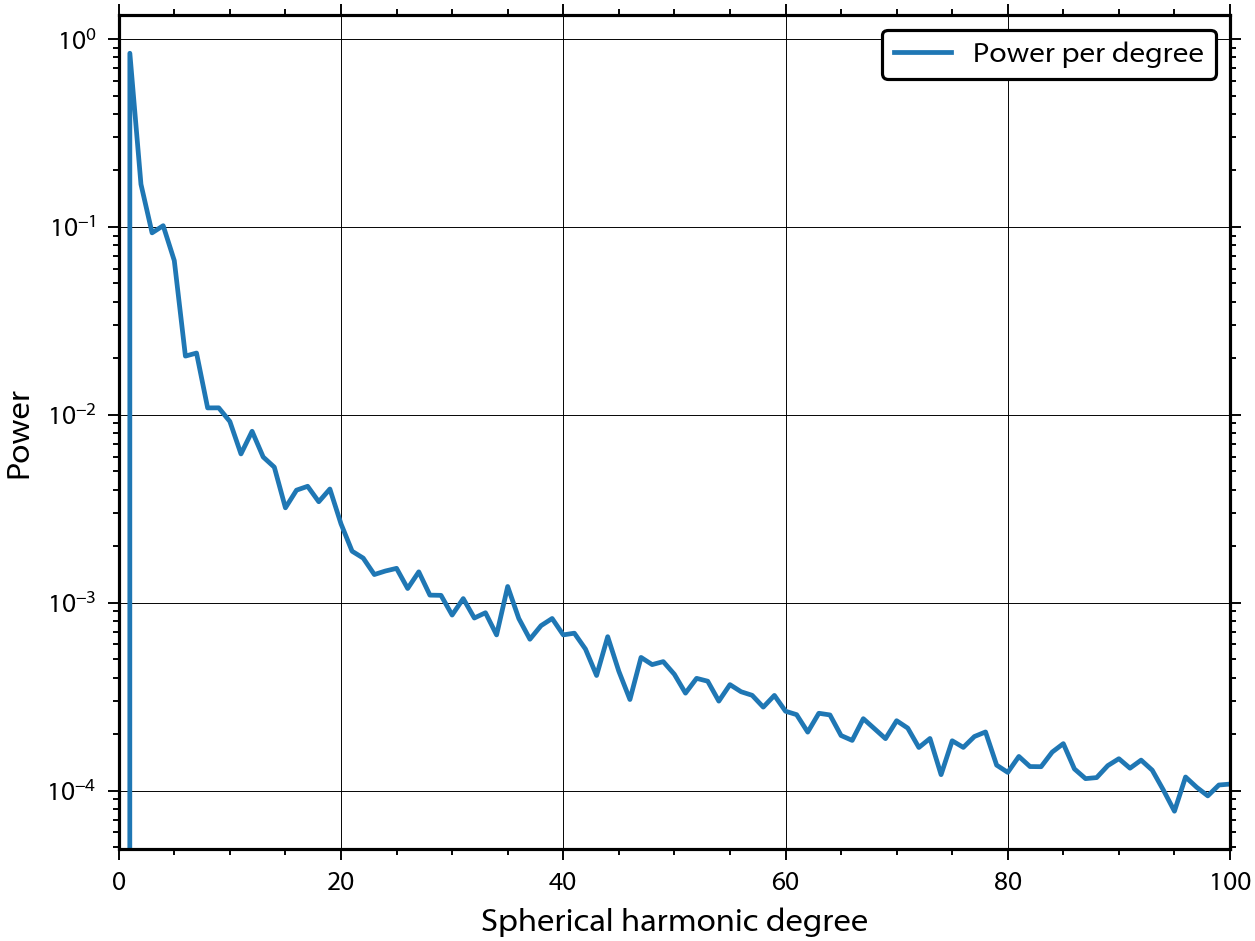By default, this method plots the power in units of power per spherical harmonic degree (per_l), but you can also choose from the average power per coefficient as a function of spherical harmonic degree (per_lm) and the power per log bandwith (per_dlogl). You can visualize the power asociated with each spherical-harmonic coefficient using the plot_spectrum2d() method. In this example, we use the optional parameter vrange to scale the colormap from 0.1 to 1.e-7 times the maximum value.

In :
fig, ax = clm.plot_spectrum2d(vrange=(1.e-7,0.1), show=False)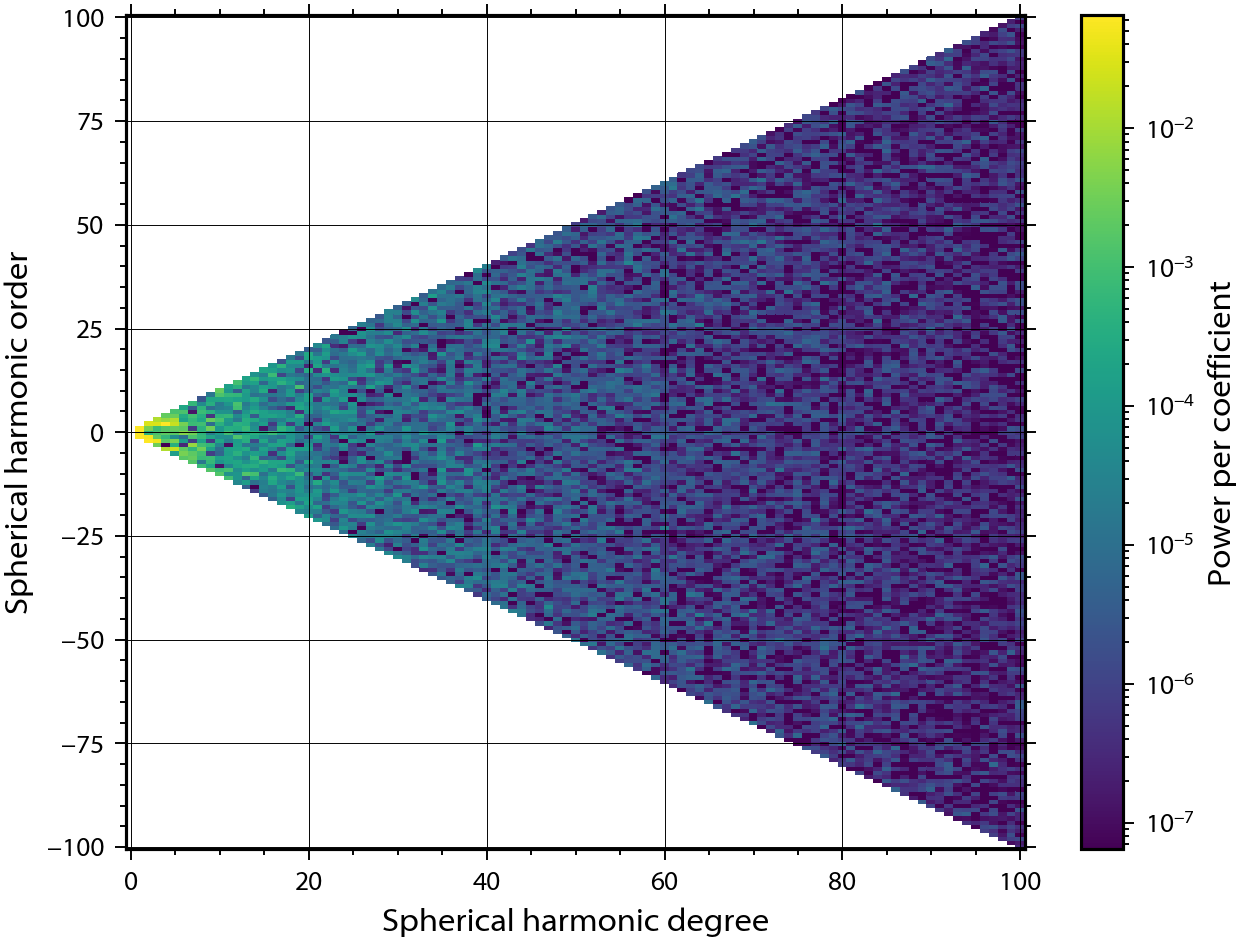The spherical harmonic coefficients of a given SHCoeffs class instance can be converted easily to a different normalization using the convert() method. Here, we will convert the coeffients to the orthonormalized convention using the Condon-Shortley phase, which is common in many fields of physics and seismology. Also, let's just return the first 50 degrees of the function:

In :
clm_ortho = clm.convert(normalization='ortho', csphase=-1, lmax=50)


If you ever forget how your data are normalized, you can use the built in info() method to remind you:

In :
clm_ortho.info()

kind = 'real'
normalization = 'ortho'
csphase = -1
lmax = 50
header = None


We will next calculate the power spectra of our two functions and plot them along with our input expectation spectrum:

In :
fig, ax = clm.plot_spectrum(legend='Orthonormalized', show=False)
clm_ortho.plot_spectrum(ax=ax, linestyle='dashed', legend='4$\pi$ normalized')
ax.plot(clm.degrees(), power, '-k')
limits = ax.set_xlim(0, 100)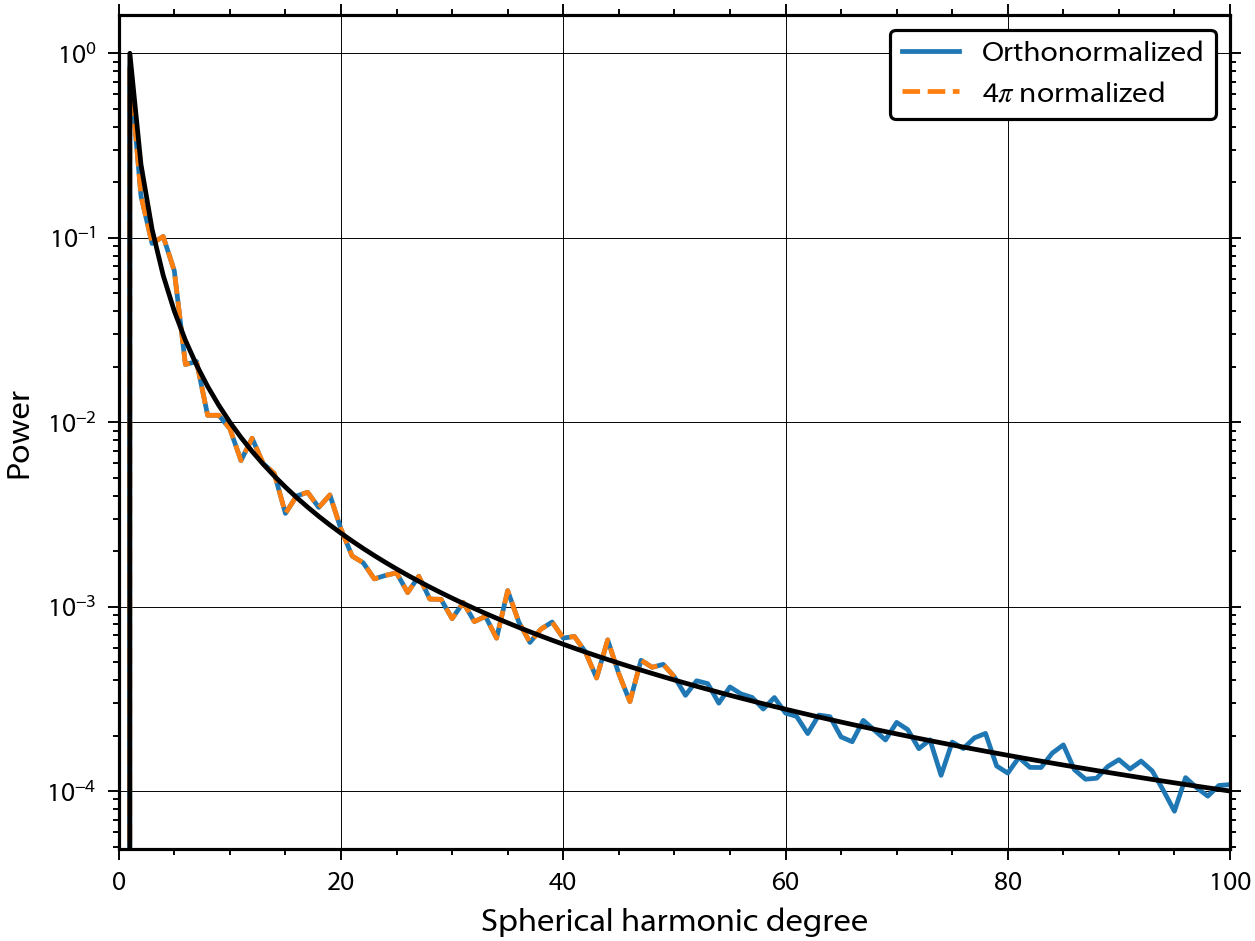The method degrees() was here used to return a numpy list of the degrees from 0 up to the maximum value of the coefficients. As you can see, the power spectrum of the random realization follows closely the input expectation spectrum. Furthermore, the power spectra are seen to be independent of the chosen normalization.

Next, let's expand the data onto a grid and plot it. We first use the expand() method, which returns a new instance of the class SHGrid, and then plot the data using the built in method plot().

In :
grid = clm.expand()
fig, ax = grid.plot(show=False)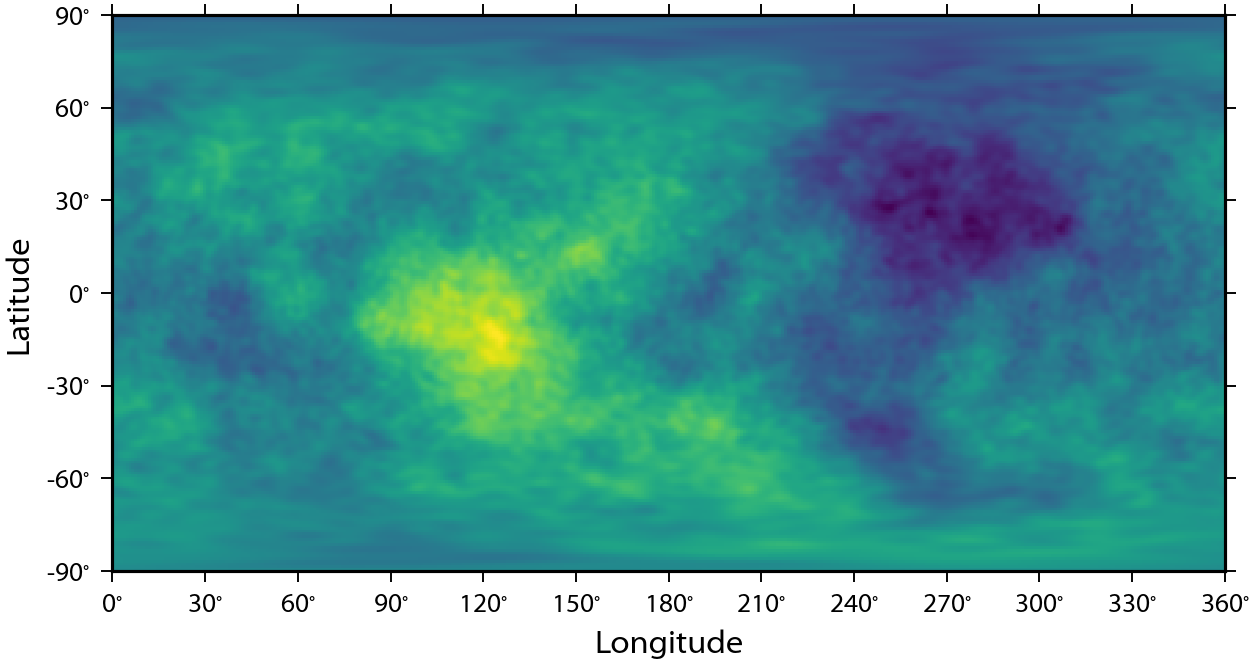pyshtools makes use of three different grid types. The default is to use grid='DH', which is an equally-sampled grid in latitude and longitude that conforms to Driscoll and Healy's (1994) sampling theorem. If you would like a grid that is oversampled in longitude by a factor or two, such that the number of longitude bands is twice that as the number of latitude bands, use grid='DH2' instead.

The third grid is constructed explicitly for use with Gauss-Legendre quadrature integration techniques. This grid contains about half as many latitude bands as an equivalent DH grid, but the latitudes are unequally sampled at the zeros of the Legendre polynomial of the maximum degree of the coefficients. The following commands show how to expand the spherical harmonic coefficents onto a GLQ grid, plot it, and output lists that contain the latitudes and longitudes (in degrees) for each row and column of the grid:

In :
grid_glq = clm.expand(grid='GLQ')
grid_glq.plot(show=False)

lats = grid_glq.lats()
lons = grid_glq.lons()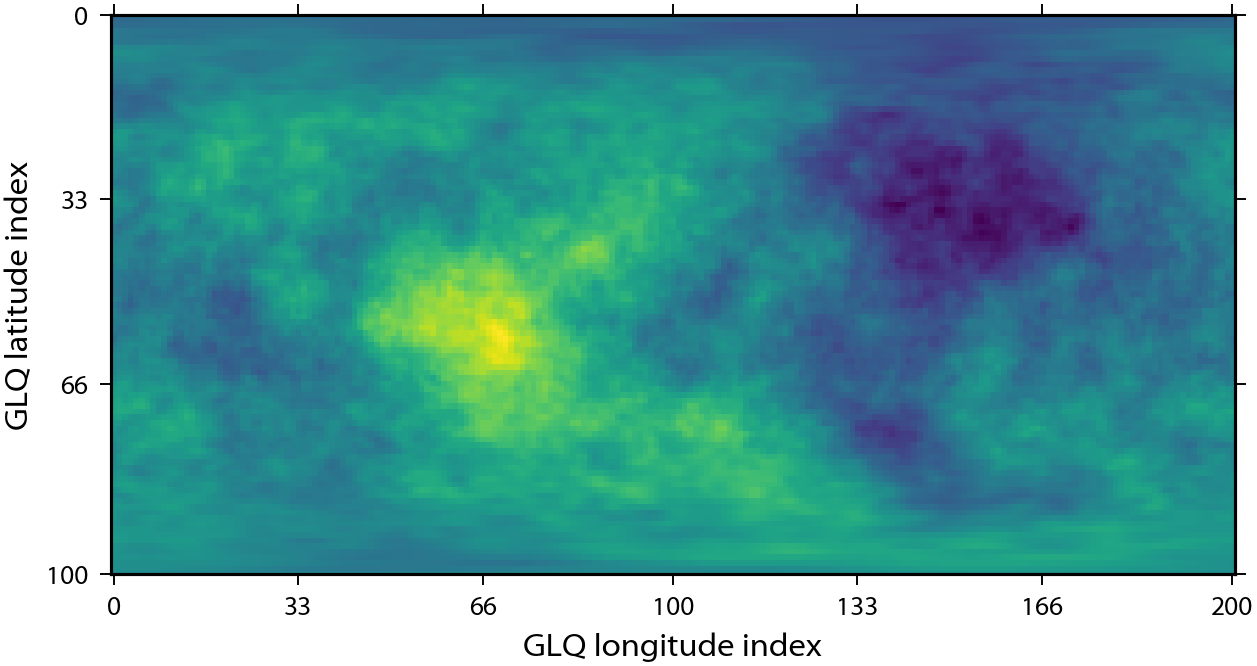If you ever forget how your grid was constructed, the info() method provides you with everything you need to know:

In :
grid_glq.info()

kind = 'real'
grid = 'GLQ'
nlat = 101
nlon = 201
lmax = 100

In :
grid.info()

kind = 'real'
grid = 'DH'
sampling = 2
nlat = 202
nlon = 404
lmax = 100


Sometimes you need to set individual spherical harmonic coefficients to a specified value. For example, for planetary topography, the degree 2 order 0 term can be atypically large as a result of the planet's rotation. Let's set this term equal to zero and replot it using an over-sampled DH grid. Though we will modify only a single coefficient here, you could also provide input arrays for l, m, and value. When using the set_coeffs() method, you set the cosine terms using positive values for the orders, and the sine terms using negative orders. We expand the modified coefficients on a grid, and then plot the data with a vertical color bar:

In :
clm.set_coeffs(values=0., ls=2, ms=0)
grid_dh2 = clm.expand(grid='DH2')
fig, ax = grid_dh2.plot(colorbar=True, cb_orientation='vertical', cb_label='My data', show=False)
grid_dh2.info()

kind = 'real'
grid = 'DH'
sampling = 2
nlat = 202
nlon = 404
lmax = 100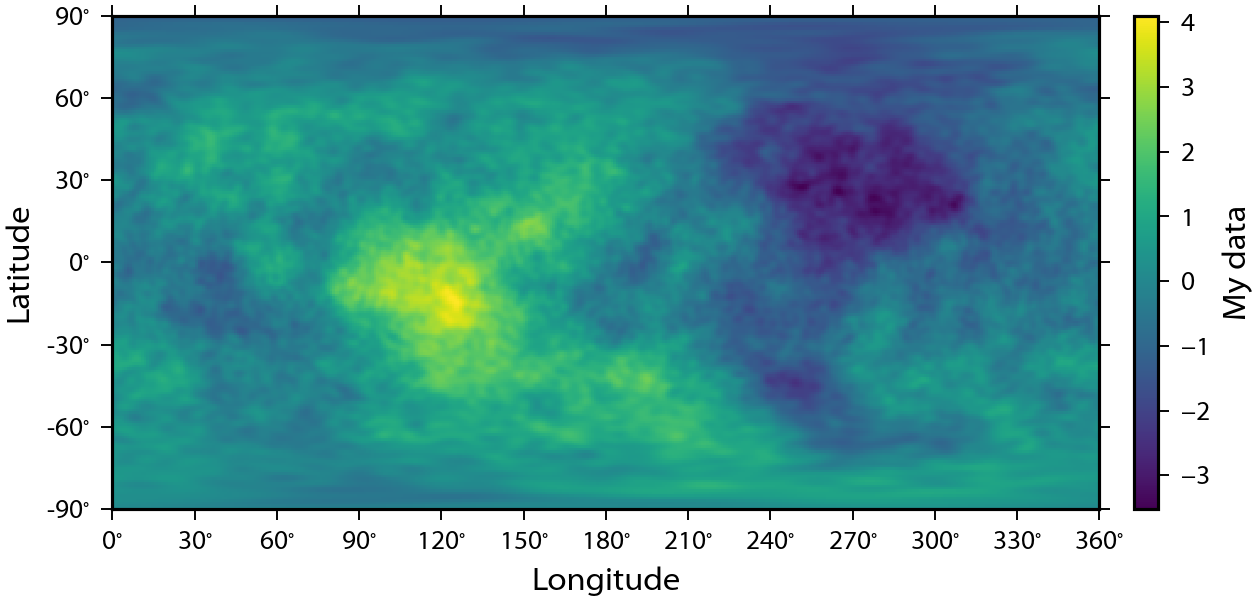If you want to save either the raw spherical harmonic coefficients to a file, or save the raw gridded data to a file, this can be done using the to_file() methods. If you want to extract the grid or coefficients as a numpy array, this can be done using the to_array() methods. Here, we will just extract the first few degrees of the spherical harmonic coefficients and verify that the coefficient was indeed set to zero:

In :
coeffs = clm.to_array(lmax=2)
print(coeffs, end='\n\n')
print('c20 = {:f}'.format(coeffs[0,2,0]))

[[[-0.          0.          0.        ]
[-0.29989808 -0.32085104  0.        ]
[ 0.          0.06300036  0.17195867]]

[[ 0.          0.          0.        ]
[ 0.          0.80448323  0.        ]
[ 0.          0.22521439 -0.28984385]]]

c20 = 0.000000


Calculating the spherical harmonic coefficients from a grid is as easy as expanding the coefficients onto a grid:

In :
clm_new = grid_dh2.expand()


If you need to evaluate the spherical harmonic coefficients at a specific set of points, this can also be accomplished using the expand() method. All that needs to be done is to provide a list or numpy array of the latitude and longitude coordinates:

In :
clm.expand(lat=[90., 10., -45., -90.], lon=[0., 90., 275., 0.])

Out:
[-1.2281625451237126,
1.4442220947502393,
-0.07626479046637802,
0.0934326306370466]

It is also easy to rotate either the physical body or the underlying coordinate frame of the function expressed in spherical harmonics. This is accomplished using three Euler angles, alpha, beta and gamma. There are many different conventions for these angles, so please read the documentation before doing this blindly! Here, we will rotate the point at the north pole to 60 degrees north latitude and 180 degrees east longitude:

In :
clat = 60.
clon = 180.

alpha = 0.
beta = -(90.-clat)
gamma = -clon

clm_rotated = clm.rotate(alpha, beta, gamma, degrees=True)
grid_rotated = clm_rotated.expand()
fig, ax = grid_rotated.plot(show=False)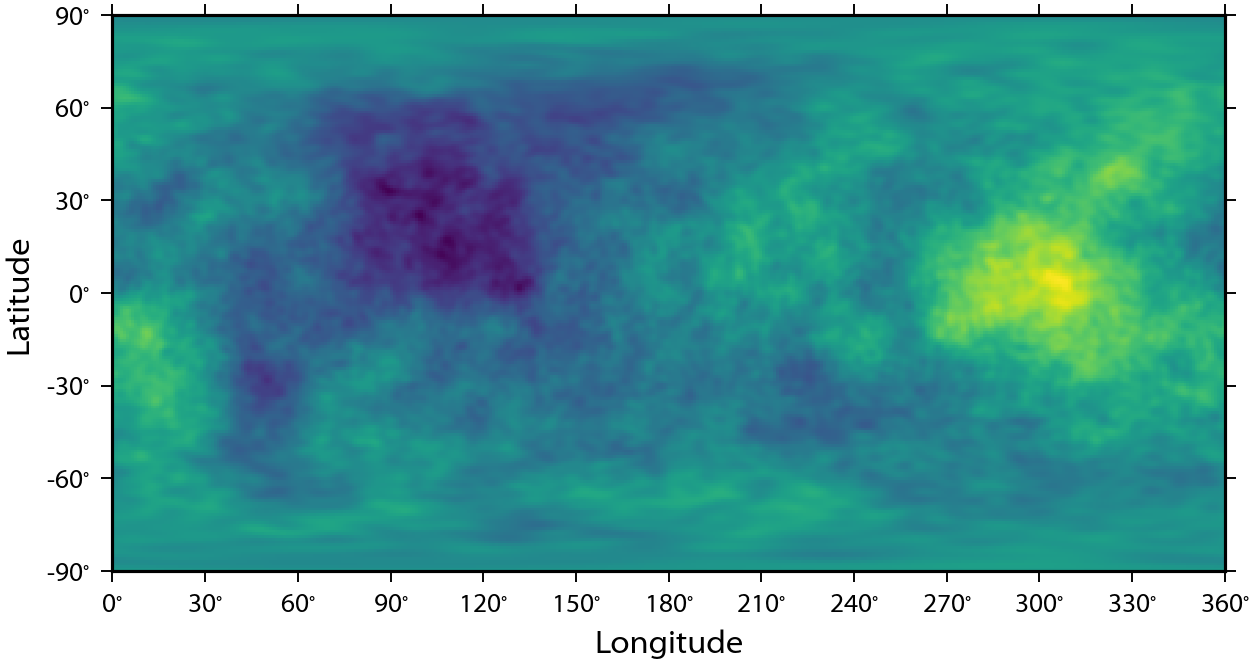By default, this routine expects the angles to be in degrees, which we specified redundantly by the provided optional parameter. If the angles were in radians, you would instead set this to False.

You can also perform simple arithmetic operations on SHCoeffs and SHGrid class instances. Let's create a second set of random coefficients whose power is 10 times less than our first set and expand this onto a grid.

In :
clm2 = pyshtools.SHCoeffs.from_random(power/10., seed=54321)
grid2 = clm2.expand()
fig, ax = grid2.plot(show=False)